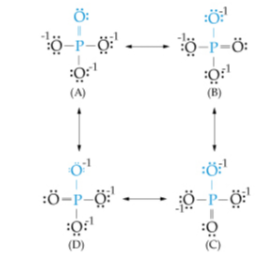# Problem: The bond order for a molecule is the number of bonds between a pair of atoms. For example, for the oxygen molecule, O = O, the bond order is two; for the hydrogen molecule, H - H, the bond order is one. Bond order indicates the strength of the bond. Consider the four resonating structures of the phosphate ion: Calculate the average bond order for a P - O bond (such as the one shown in blue) in a phosphate ion. Express your answer numerically as a decimal or improper fraction.

###### FREE Expert Solution
• For this problem, we are asked for the average bond order of P-O bond

• From the paragraph, it tells us that a single bond is equal to bond order of 1 while 2 bonds/double bonds is equal to bond order of 2###### Problem Details

The bond order for a molecule is the number of bonds between a pair of atoms. For example, for the oxygen molecule, O = O, the bond order is two; for the hydrogen molecule, H - H, the bond order is one. Bond order indicates the strength of the bond. Consider the four resonating structures of the phosphate ion: Calculate the average bond order for a P - O bond (such as the one shown in blue) in a phosphate ion. Express your answer numerically as a decimal or improper fraction.